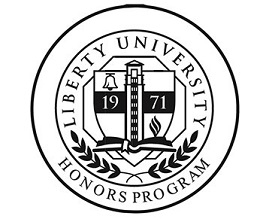## Senior Honors Theses

Spring 4-15-2015

#### School

College of Arts and Sciences

Mathematics

#### Keywords

Symmetry, Differential Forms, Lie Groups, ODE, Lie Derivative, Group, Differential Equations, Ordinary Differential Equations, Ordinary

#### Disciplines

Algebra | Geometry and Topology | Ordinary Differential Equations and Applied Dynamics | Other Mathematics

#### Abstract

Differential equations have bearing on practically every scientific field. Though they are prevalent in nature, they can be challenging to solve. Most of the work done in differential equations is dependent on the use of many methods to solve particular types of equations. Sophus Lie proposed a modern method of solving ordinary differential equations in the 19th century along with a coordinate free variation of finding the infinitesimal generator by combining the influential work of Élie Cartan among others in the field of differential geometry. The driving idea behind using symmetries to solve differential equations is that there exists a coordinate system for any given differential equation such that the equation can be directly integrated within the newfound system.

COinS